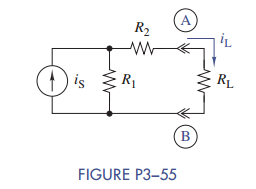### Create an Account

Home / Questions / (a) Find the Thévenin or Norton equivalent circuit seen by RL in Figure P3−55. (b) Use the...

# (a) Find the Thévenin or Norton equivalent circuit seen by RL in Figure P3−55. (b) Use the...

(a) Find the Thévenin or Norton equivalent circuit seen by RL in Figure P3−55.

(b) Use the equivalent circuit found in part (a) to find iL in terms of iS, R1, R2, and RL.Jul 28 2020 View more View LessSubscribe To Get Solution